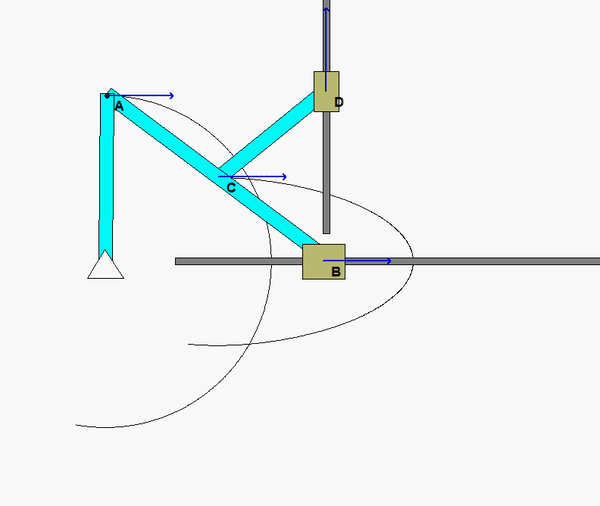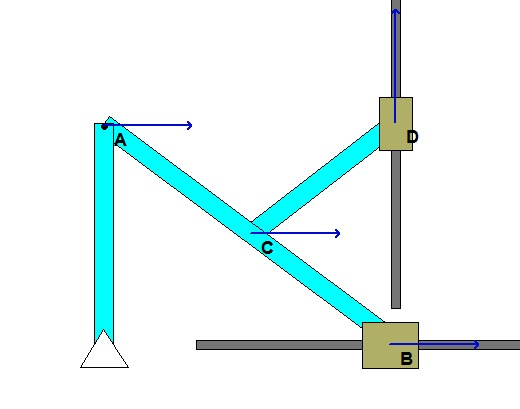# Homework H2.H - Sp23

 Problem statement Solution videoDiscussionThe animation above shows the motion of the mechanism over a range of input angles of link OA. For a given position, envision the location of the instant centers (ICs) for links AB and CD. Do the directions and magnitudes for the velocities of points B, C and D agree with the location of these ICs?

Shown below if a freeze-frame of the mechanism motion at the position for which you are asked to do analysis. From this figure, where are the two ICs for links CD and BC? In particular, how does the position of the IC for AB relate to the relative sizes of the speeds of points A, C and B? What is the angular velocity of link AB at this position?## 28 thoughts on “Homework H2.H - Sp23”

1.Myles Christian Kappes says:

Is there meant to be a numeric value for Woa? I am unsure on how to calculate the speed of other points without.

2.CMK says:

3.Jackson Charles Miller says:

I'm confused about how to proceed if IC_ab is infinity. How would we find V_B?

1.James Jung says:

I also got IC_ab as infinity, but that would mean that V_a = L*omega_OA = V_b. I would still be stuck on how to find V_c.

2.CMK says:

If the IC for a link is at infinity, then the angular velocity of the link is zero. If the angular velocity for link is zero, then all points on the link have the same velocity, in both magnitude and direction.

1.James Jung says:

Based on the freeze-frame, v_D is perpendicular to v_C=v_A=v_B but how would that relate to determine v_D.

1.CMK says:

Construct perpendiculars to v_C and v_D. The intersection of these two perpendiculars locates the IC for CD. Since you know v_C, you can use that location of the IC to determine the angular speed of CD, as well as v_D.

4.J Cena says:

If a link is pinned to a point that would make it automatically its instant center correct?

1.CMK says:

That is correct.

5.J Cena says:

Is the velocity at each point on a rigid body equal when w=0?

1.Niklas Eric Anderson says:

When w=0 the body is in pure translation, meaning that all points along that rigid body are moving at the same speed in the same direction. This can be seen in the Lecture Book examples on page 107.

1.Drew Thomas Casterton says:

At first, I thought since there were non-zero velocities to the links omega couldn't be zero. I guess remembering that omega is rotational velocity and not just a velocity value is important. Hopefully this gives a bit of an intuitive 'spin' to what Niklas said.

2.CMK says:

Yes, that is true.

6.Michael Bradley Mayhew says:

When looking at the distance from C to IC of CD, would that distance equal half of OA? The image looks proportional to me, and by eye it looks like C is roughly at the midpoint of OA in the vertical plane. If not, how do we find the distance from C or D to the IC?

1.CMK says:

Michael: Consider the right triangle AOB. Point C is at the midpoint of the hypotenuse of the triangle. Therefore, its vertical position is at half of 0.6L up from the base, and is half of 0.8L along the base.

Does this help?

1.Michael Bradley Mayhew says:

Yes that helps a lot! I was able to solve for it from there. Just to confirm, in my new Vc and Vd equations, the distance I use is the distance relative to the IC correct? I used .3L from C to the IC, then .4L from D to the IC.

1.CMK says:

Michael: Yes, that is correct.

7.Allie says:

I don't understand how to find omega_CD so that you can in turn find v_D. How can I find this value?

1.Mason James Fellwock says:

I believe that Va, Vb, and Vc have the same magnitude and direction because their IC goes to infinity. This means that you would use the equation wCD=r*Vc

1.CMK says:

Mason: I think that you meant vC = r*wCD.

2.Elijah Allan Collins says:

You can find omega_CD by using your known value of Vc. You can use it in the equation Vc = omega_CD*r(from C to your IC). Since we know the distance from C to the IC for CD, you can solve for omea_CD. Then you use that to find VD. Let me know if I messed that up at all. Thanks!

8.Mason James Fellwock says:

I am still a little confused, if wOA is zero and we are trying to calculate Va, are we supposed to still use the w=r*V equation?

1.Niklas Eric Anderson says:

Mason, be sure that you aren’t mixing up the rotational velocity of the rigid body AB and the rotational velocity of point A. These two values are distinct, and you can use the w = r * V equation for point A.

2.CMK says:

omega_OA is given. I do not think that omega_OA = 0. Furthermore, the equation goes as: v = r*w.

9.Adam Jerry Walker says:

I am a little confused on what the instant center for beam BA is supposed to be as the perpendicular component to the velocity is just infinity.

1.CMK says:

Not exactly sure of what you are saying here. Since the IC for AB is at infinity, ALL points on AB have the same velocity; that is, v_A = v_B = v_C (in both magnitude and direction).

10.Zachary William Delahunty says:

For part A, when it asks for the direction of pin C, can we leave it in terms of i,j,k vectors?

1.CMK says:

Yes, give the direction of motion for C in terms of i and j components. There will not be any components in the k direction.